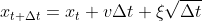# Hitting time probabilities for latent diffusion processes

Hi everyone,

I’m trying to implement a drift diffusion model in pyro, and I’m having trouble figuring out what the best way to get hitting time probabilities is. The model is made up of a latent diffusion process ‘x’ that evolves according to a drift rate ‘v’ and gaussian white noise (xi):This drift process continues until ‘x’ hits an upper or lower threshold. Once it does, a response is emitted after a certain delay (parameterized by ’t_err’ below). The distance between these two thresholds is parameterized by ‘a’. (visualization of the whole process)

My data is a collection of these emissions – -1s or 1s, corresponding to the upper or lower boundary being hit, as well as the time it took for this whole process to reach the boundary. My goal right now is to infer the drift rate, boundary separation, and delay period based on this data (‘v’, ‘a’, and ’t_err’). Eventually, I’d like to turn this into a hierarchical model and add some extra terms to the drift process.

At the moment my model looks like this:

``````def random_walk_m(emission_times, bound_hit, dt = 0.005) :
v = pyro.sample('v', dist.Normal(3, 1))
a = pyro.sample('a', dist.Normal(3, 1))
t_er = pyro.sample('t_er', dist.Gamma(.4, 2))
#calculate the time spent in the diffusion process.
t_effective = torch.nn.functional.relu(
((emission_times - t_er) / dt) - 1).long()  #subtract one to use this value for slicing below
rt_dim = (emission_times.max() / dt).int() #maximum amount of time it took for the process to hit the boundary
trls = emission_times.shape
with pyro.plate('b', trls) as ind:
steps = pyro.sample('steps', dist.Normal(v * torch.ones(rt_dim) * dt,
math.sqrt(dt)).to_event(1),
infer = {'enumerate' : 'parallel',
'expand' : True, 'num_samples' : 10}) #marginalize out the latent drift process

walk = pyro.deterministic('walk', steps.cumsum(dim = -1))
out = pyro.sample('out', dist.Normal(walk[..., ind, t_effective] / a, .0001),
obs = bound_hit[ind])
#out = pyro.sample('out', dist.Normal(walk[..., ind, t_effective], 1),
# obs = bound_hit[ind] * a)
``````

The idea is to use ‘t_er’ to calculate the amount of time spent in the drift process, ‘v’ to generate random walks for each of my data points, and ‘a’ to express the value of the drift process relative to the boundary. There’s a lot going wrong here… From what I can tell these are my main problems:

• I’m not modelling the first hitting time probability. That is, I’m not representing the probability that the first time the drift process hits one of the two boundaries is at t_effective’.
• I can’t figure out a principled way to form the sample statement for the ‘out’ variable – it feels pretty arbitrary to use a normal distribution for this.
• Using ‘t_er’ to generate a long tensor seems wrong with respect to gradients.
• This runs too slowly to scale to a hierarchical model.
• Not sure if this is a problem but I get a `RuntimeWarning: Site steps is multiply sampled in model` when using SVI.

With all that said, I wanted to ask you all what you think the best way to model my problem would be. Is there any way to either salvage the model above, or use an HMM-style approach to solve these problems – i.e. ‘unrolling’ my data into sequences of 0s until a response is recorded at which point I’d code the responses with 1s or 2s? I’ve tried the latter approach, but I couldn’t figure out how to incorporate ‘t_er’ and ‘a’ into the model…

Here’s a full script :

``````import pyro
import numpy as np
pyro.enable_validation(True)
from pyro.infer import MCMC, NUTS
from pyro import poutine
import pyro.distributions as dist; from pyro.optim import Adam
from pyro.infer import SVI, JitTraceEnum_ELBO; from pyro import poutine
from pyro.contrib.autoguide import AutoDelta
import torch
import math

def drift_diffusion(v, a, t_er, sigma = 1, tmax = 100, dt = 0.005) :
time = np.arange(dt, tmax + 1, dt)
dX = v * dt + sigma * np.sqrt(dt) * np.random.randn(len(time))
X = np.cumsum(dX)
val = np.argwhere(np.abs(X) >= a) #time steps it took to reach boundary
if X[val] >= a :
val = val*dt + t_er
else :
val = (val * dt + t_er) * -1
return val
def rt_sim(v, a, t_er, tmax = 2000, dt = 0.005, steps = 400) :
'''simulate n trials'''
rt = np.zeros(steps)
for i in range(steps) :
rt[i] = drift_diffusion(v, a, t_er, dt = dt)
return rt

times = rt_sim(1, .5, .2, dt = 0.005, steps = 1500)
#plt.hist(times, bins = 50) ; plt.show()

choices = torch.tensor([1 if x > 0 else -1 for x in times], dtype = torch.float)
timings = torch.tensor(np.abs(times), dtype = torch.float)
data = torch.stack((timings, choices), dim= -1)

def random_walk_m(emission_times, bound_hit, dt = 0.005) :
v = pyro.sample('v', dist.Normal(3, 5))
a = pyro.sample('a', dist.Normal(3, 5))
t_er = pyro.sample('t_er', dist.Gamma(.4, 2))
t_effective = torch.nn.functional.relu(
((emission_times - t_er) / dt)).long() - 1
#calculate the time spent in the diffusion process. subtract one for indexing later on
rt_dim = (emission_times.max() / dt).int()
trls = emission_times.shape
with pyro.plate('b', trls) as ind:
steps = pyro.sample('steps', dist.Normal(v * torch.ones(rt_dim) * dt,
math.sqrt(dt)).to_event(1),
infer = {'enumerate' : 'parallel',
'expand' : True, 'num_samples' : 10})

walk = pyro.deterministic('walk', steps.cumsum(dim = -1))
out = pyro.sample('out', dist.Normal(walk[..., ind, t_effective] / a, .0001),
obs = bound_hit[ind])

kernel = NUTS(random_walk_m, jit_compile = True, ignore_jit_warnings = False)
sample = MCMC(kernel,
num_samples = 3000,
warmup_steps = 3000,
num_chains = 1,
mp_context= 'fork')
sample.run(timings, choices)

pyro.clear_param_store()
adam_params = {"lr": 0.005, "betas": (0.9, 0.99)}
guide = AutoDelta(poutine.block(random_walk_m,
hide_fn = lambda msg : msg["name"].startswith('steps')))
svi = SVI(random_walk_m, guide, optimizer, loss=JitTraceEnum_ELBO())
losses = []
steps = 1500
for step in range(steps):
losses.append(svi.step(timings, choices))
if step % 300 == 0:
print(f'at step {step}')
``````

Lastly, I just wanted to mention that I’ve really been really enjoying learning pyro! It’s a great framework, and I’m looking forward to going deeper with it.

Summary

This text will be hidden

1 Like